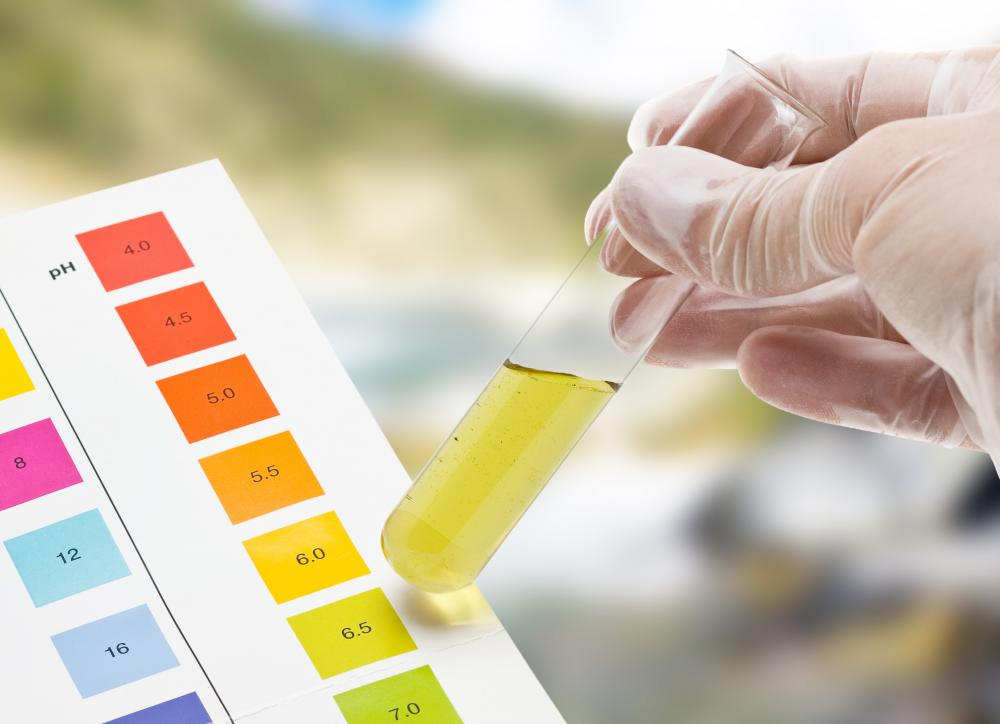# What Is the Connection between pH and Concentration?

Richard Nelson

Perhaps the most important connection between pH and concentration is that pH is by its very nature a measure of the concentration of hydrogen ions in a given solution. Compounds with high pH readings are basic or alkaline, whereas those with lower readings are acidic. Readings vary between zero and fourteen, with the average, seven, being neutral pH. The properties of acids and bases are determined by both pH and the concentration of the acid and base molecules in a solution. As such, the two concepts necessarily go hand in hand. It is difficult to talk about a given solution’s pH without some understanding of the concentration of elements it contains, and knowing the concentration breakdown of certain compounds necessarily sheds light on its pH. Chemists and researchers typically calculate the two together.The pH changes based on the concentration of hydrogen ions in a substance.

#### Understanding pH Generally

Broadly speaking, pH is a measurement of the concentration of hydrogen ions in any given solution. It has been widely suggested that the letters “p” and “h” stand for “power of hydrogen,” though this is not firmly established in the scientific community. What is established, though, is the universal importance of pH; as a measurement it is used in all sorts of chemical and mathematical applications, and is important to a wide variety of processes, from food manufacturing to drug creation.

#### On a Logarithmic Scale

All solutions have a specific pH and are made with a solvent, usually water, and a solute, which dissolves easily in the chosen solvent. Using a logarithmic scale for pH helps keep the scale small while retaining the valuable information the pH scale represents. One connection between pH and the concentration of hydrogen ions is that together they allow the use of a logarithmic scale. The lower the pH the more concentrated the hydrogen ions, because pH measures the negative log of hydrogen ion concentration, meaning 0.1 ion concentration is one on the pH scale and 0.001 is three.

Another way of thinking about this is that pH and concentration become linearly connected by a factor of ten and the pH changes by a factor of one when hydrogen ion concentration changes by a factor of ten. The normal relationship is said to be exponential and this connection helps avoid tedious equations involving higher levels of mathematics. This automatically changes the curved relationship to a linear relationship where simple math applies. The straight line can make predictions, relationships between different concentrations, and calculations easy to visualize.

#### Calculation Similarities

Another connection between pH and concentration is that both are calculated in a similar way. Solution concentration measures how much of a certain atom or molecule is present compared to the entire solution. The pH is a special concentration measurement that considers how many hydrogen ions are in a solution compared to everything else. Most other concentration calculations do not use the pH scale. Many use a unit called Molarity, which is the amount of atoms or molecules available per liter of solvent.